# What is an activation function?

An activation function is a very important feature of an artificial neural network , they basically decide whether the neuron should be activated or not

So lets consider an simple neural network shown below.

In the above figure,(x1,x2,…xn)is the input signal vector that gets multiplied with the weights(w1,w2,…wn). This is followed by accumulation ( i.e. summation + addition of bias b). Finally, an activation function f is applied to this sum.

# Why do we use an activation function in neural network ?

As observed for the above figure when we do not have the activation function the weights and bias would simply do a linear transformation.

A linear equation is simple to solve but is limited in its capacity to solve complex problems and have less power to learn complex functional mappings from data. A neural network without an activation function is just a linear regression model.

The activation function does the non-linear transformation to the input making it capable to learn and perform more complex tasks. We would want our neural networks to work on complicated datas like videos , audio , speech etc. Linear transformations would never be able to perform such tasks.

# What condition the activation function should satisfy?

Activation functions make the back-propagation possible since the gradients are supplied along with the error to update the weights and biases. Without the differentiable non linear function, this would not be possible.

So the functions should be differentiable and monotonic.

Derivative or Differential: Change in y-axis w.r.t. change in x-axis.It is also known as slope.

Monotonic function: A function which is either entirely non-increasing or non-decreasing.

# Types of activation functions?

The Activation Functions can be basically divided into 2 types-

1. Linear or Identity Activation Function
2. Non-linear Activation Functions

As you can see the function is a line or linear.Therefore, the output of the functions will not be confined between any range.

Equation : f(x) = x

Range : (-infinity to infinity)

As shown in the above figure the activation is proportional to the input. . This can be applied to various neurons and multiple neurons can be activated at the same time. Now, when we have multiple classes, we can choose the one which has the maximum value. But we still have an issue here

The derivative of a linear function is constant i.e. it does not depend upon the input value x.

This means that every time we do a back propagation, the gradient would be the same. And this is a big problem, we are not really improving the error since the gradient is pretty much the same. And not just that suppose we are trying to perform a complicated task for which we need multiple layers in our network. Now if each layer has a linear transformation, no matter how many layers we have the final output is nothing but a linear transformation of the input.

The Nonlinear Activation Functions are the most used activation functions.It makes it easy for the model to generalize or adapt with variety of data and to differentiate between the output.

The Nonlinear Activation Functions are mainly divided on the basis of their range or curves-

1. Sigmoid or Logistic Activation Function

The Sigmoid Function curve looks like a S-shape.

Equation : f(x) = 1 / 1 + exp(-x)

Range : (0 to 1)

Pros:

1.The function is differentiable.That means, we can find the slope of the sigmoid curve at any two points

2.The function is monotonic but function’s derivative is not

Cons:

1.It gives rise to a problem of “vanishing gradients”, since the Y values tend to respond very less to changes in X

2.Secondly , its output isn’t zero centered. It makes the gradient updates go too far in different directions. 0 < output < 1, and it makes optimization harder.

3. Sigmoids saturate and kill gradients.

4. Sigmoids have slow convergence.

2. Tanh or hyperbolic tangent Activation Function:

Equation : f(x) = 1 — exp(-2x) / 1 + exp(-2x) or 2 *sigmoid(2x)-1

Range : (-1 to 1)

Pros:

1. The function and its derivative both are monotonic
2. Output is zero centered
3. Optimization is easier

Cons:

1. It also suffers vanishing gradient problem
2. It saturate and kill gradients.

3. ReLU (Rectified Linear Unit) Activation Function

The ReLU is the most used activation function in the world right now

Equation : f(x) = max(0,x)

Range : (0 to infinity)

Pros:

1. The function and its derivative both are monotonic.
2. Due to its functionailty it does not activate all the neuron at the same time
3. It is efficient and easy for computation.

Cons:

1. The outputs are not zero centered similar to the sigmoid activation function
2. When the gradient hits zero for the negative values, it does not converge towards the minima which will result in a dead neuron while back propagation.

4. Leaky ReLU

To solve the ReLU problem we have leaky ReLU

Equation : f(x) = ax for x<0 and x for x>0

Range : (0.01 to infinity)

Pros:

1. The function and its derivative both are monotonic
2. It allows negative value during back propagation
3. It is efficient and easy for computation.

Cons:

1. Results are not always consistent
2. During the front propagation if the learning rate is set very high it will overshoot killing the neuron

The idea of leaky ReLU can be extended even further. Instead of multiplying x with a constant term we can multiply it with a hyperparameter which seems to work better the leaky ReLU. This extension to leaky ReLU is known as Parametric ReLU.

5. Softmax

The softmax function is also a type of sigmoid function but it is very useful to handle classification problems having multiple classes .

The softmax function is shown above, where z is a vector of the inputs to the output layer (if you have 10 output units, then there are 10 elements in z). And again, j indexes the output units, so j = 1, 2, …, K.

The softmax function is ideally used in the output layer of the classifier where we are actually trying to attain the probabilities to define the class of each input.

# Which activation function to use ?

From the above we have seen different categories of activation functions, we need some logic / heuristics to know which activation function has to be should be used in which situation.

Based on the properties of the problem we might be able to make a better choice for easy and quicker convergence of the network.

• Sigmoid functions and their combinations generally work better in the case of classification problems
• Sigmoids and tanh functions are sometimes avoided due to the vanishing gradient problem
• Tanh is avoided most of the time due to dead neuron problem
• ReLU activation function is widely used as it yields better results
• If we encounter a case of dead neurons in our networks the leaky ReLU function is the best choice
• ReLU function should only be used in the hidden layers

In this article, I tried to describe the activation functions commonly used . There are other activation functions too, but the general idea remains the same. Hope this article serves the purpose of getting idea about the activation function , why when and how to use it for a given problem statement

--

--

--

Love podcasts or audiobooks? Learn on the go with our new app.

## Applying Simple Deep Learning method to Korea Historical Stock Market Data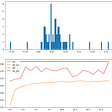## How to Improve a Machine Learning Algorithm: Regularization## A Ride in Life of A Machine learning Engineer 🧑‍💻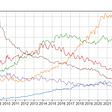## Most Effective Way To Implement Radial Basis Function Neural Network for Classification Problem## Neural Networks : Learning How To Build An Image Classification Model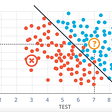## Supervised Machine Learning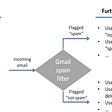## 5 Reasons Why PLCs Drive Billion Dollar Markets## Introduction to Super-Resolution Machine Learning Models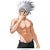## CNN for Autonomous Driving## Activation functions in machine learning## Neural Network Basics: What is an Artificial Neural Network?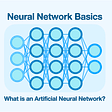## Activation Functions